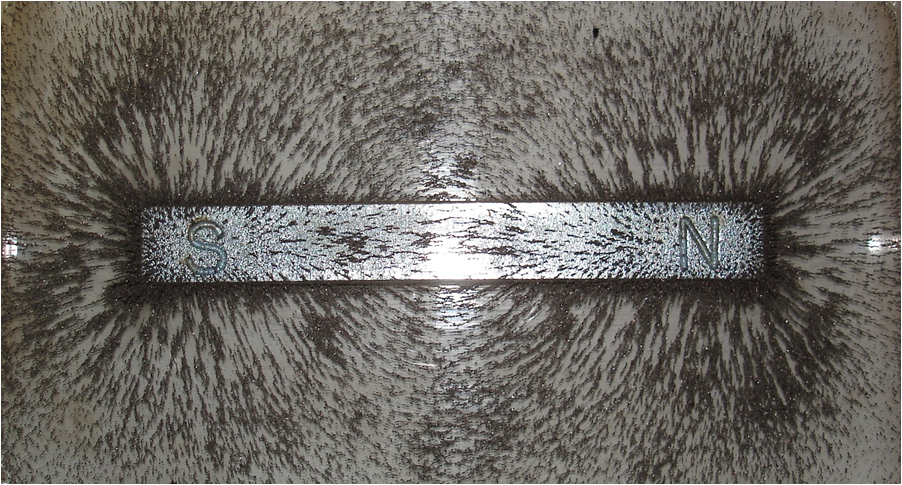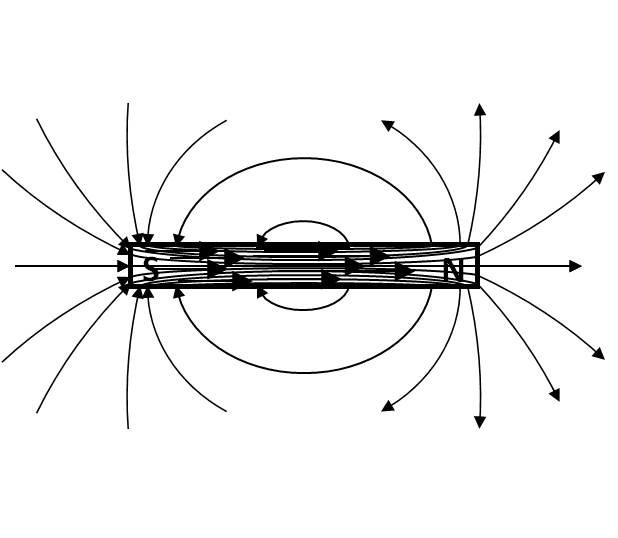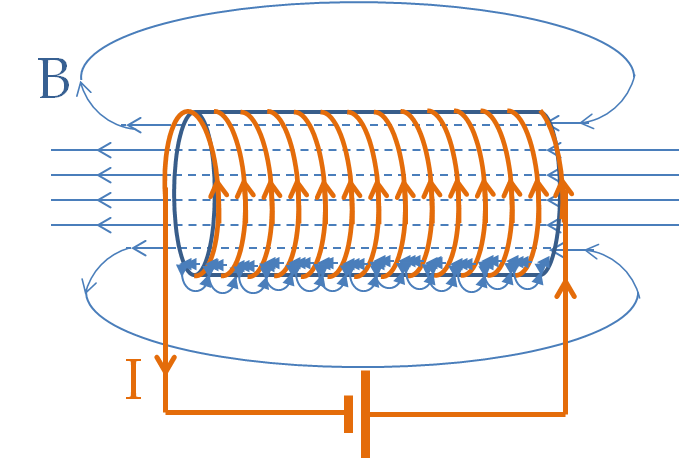## Section38.3Existence of Magnetic Field

The magnetic force between magnets act at a distance. It seems unreasonable how force propagates between magnets without anything between them. Michael Faraday came up with an alternative explanation. He imagined that each magnet sets up a field in space around it. When you place another magnet in that field, it just responds to the field at its own location. A field picture brings advantage of local description of a force.

This is similar to the field-based explanation of the action-at-distance behavior of electric and gravitational forces. However, unlike electric and gravitational field, you can easily visualize the magnetic field of a magnet by sprinkling iron filings near the magnet as shown in Figure 38.3.1. The iron particle get magnetized in a magnetic field and stick together along magnetic field lines as seen in the picture.Figure 38.3.1. Magnetic field lines visualized by sprinkling iron particles near a magnet.

Magnetic field will be denoted by $\vec B \text{.}$ You can determine the directions of magnetic field vectors in space by placing magnetic compass at space points near the magnet. We will discuss a method for finding the magnitude of the magnetic field in the next section.

When you connect the magntic field directions, we get magnetic field lines. Outside a magnet, magnetic field likes come out of the North pole and land on the South pole as illustrated for a bar magnet in Figure 38.3.2. But inside the magnet, they must continue since there are no magnetic monopoles. That means, inside a magnet the direction of magnetic field lines go from the South pole to the North pole.Figure 38.3.2. Magnetic field lines of a bar magnet. Note how field lines continue inside the magnet. Magnetic field lines make closed loops, which is one major difference with the electric field lines. This is due to a lack of existence of magnetic monopoles, i.e., North and South poles do not exist by themselves.

When drawing magnetic field lines, we represent strength of the magnetic field in the region by drawing sufficient number of lines so that the “density of lines” corresponds to the strength of the magnetic field in the region. This is similar to how we represent strength of electric field in electric field lines. Thus, the density of lines is higher near the poles of a magnet than far away from the poles, and it is highest inside the magnet since all lines must pass through the magnetic body.

When magnetic field lines are straight, then the direction of the lines are the direction in which a magnetic needle will point in the direction of South-to-North when placed at that point. If the lines are curved, then the tangent to magnetic field lines gives us the direction in which a tiny magnetic needle will point if placed there.

### Subsection38.3.1Magnetic Field of Current Carrying Wire

In April of 1820 Hans Christian Øersted of the University of Copenhagen discovered that electric currents also exert magnetic force on magnetic needles. Actually, you can also demonstrate that one current carrying wire exerts force on another current carrying wire, neither of which are magnetic material, as we will see below.

The magnetic force on a magnet by a current carrying wire is indistinguishable from the magnetic force of another magnet. Therefore, to understand the magnetic force of a current carrying wire, we say that electric current has magnetic field just like the magnetic field of other magnets.

The effect of current in a straight wire was shown to align magnetic needles such that they pointed along circles. This says that the magnetic field lines of a straight wire with a current are circles around as shown in Figure 38.2.4. These fields are not like that of a field of a bar magnet. Instead, magnetic field of coils of wire carrying current is more like the magnetic field of a bar-magnet as shown in Figure 38.3.3.Figure 38.3.3. Magnetic field lines of current through coils of wire. You can compare the magnetic field lines here to that of a bar magnet. In the present configuration, the analogy shows that right side of the coil acts as “South Pole” and the left side as “North Pole” of a temporary magnet.

The magnetic field of current through coils is straight near the middle of the loops. If the coils are tightly wound, then the magnetic field on the sides are nearly zero and one obtains uniform magnetic field inside the space enclosed by the coils. You can try to see how these magnetic field lines remind you of fields of a bar magnet and help explain the attraction and repulsion of magnet shown in Figure 38.2.5.